# 7.5 Graphing equations in slope-intercept form

 Page 1 / 1
This module is from Elementary Algebra by Denny Burzynski and Wade Ellis, Jr. In this chapter the student is shown how graphs provide information that is not always evident from the equation alone. The chapter begins by establishing the relationship between the variables in an equation, the number of coordinate axes necessary to construct its graph, and the spatial dimension of both the coordinate system and the graph. Interpretation of graphs is also emphasized throughout the chapter, beginning with the plotting of points. The slope formula is fully developed, progressing from verbal phrases to mathematical expressions. The expressions are then formed into an equation by explicitly stating that a ratio is a comparison of two quantities of the same type (e.g., distance, weight, or money). This approach benefits students who take future courses that use graphs to display information.The student is shown how to graph lines using the intercept method, the table method, and the slope-intercept method, as well as how to distinguish, by inspection, oblique and horizontal/vertical lines. This module contains an overview of the chapter "Graphing Linear Equations and Inequalities in One and Two Variables".

## Overview

• Using the Slope and Intercept to Graph a Line

## Using the slope and intercept to graph a line

When a linear equation is given in the general form , $ax+by=c$ , we observed that an efficient graphical approach was the intercept method. We let $x=0$ and computed the corresponding value of $y$ , then let $y=0$ and computed the corresponding value of $x$ .

When an equation is written in the slope-intercept form , $y=mx+b$ , there are also efficient ways of constructing the graph. One way, but less efficient, is to choose two or three $x\text{-values}$ and compute to find the corresponding $y\text{-values}$ . However, computations are tedious, time consuming, and can lead to errors. Another way, the method listed below, makes use of the slope and the $y\text{-intercept}$ for graphing the line. It is quick, simple, and involves no computations.

## Graphing method

1. Plot the $y\text{-intercept}$ $\left(0,\text{\hspace{0.17em}}b\right)$ .
2. Determine another point by using the $\text{slope}\text{\hspace{0.17em}}m$ .
3. Draw a line through the two points.

Recall that we defined the $\text{slope}\text{\hspace{0.17em}}m$ as the ratio $\frac{{y}_{2}-{y}_{1}}{{x}_{2}-{x}_{1}}$ . The numerator ${y}_{2}-{y}_{1}$ represents the number of units that $y$ changes and the denominator $x_{2}-x_{1}$ represents the number of units that $x$ changes. Suppose $m=\frac{p}{q}$ . Then $p$ is the number of units that $y$ changes and $q$ is the number of units that $x$ changes. Since these changes occur simultaneously, start with your pencil at the $y\text{-intercept}$ , move $p$ units in the appropriate vertical direction, and then move $q$ units in the appropriate horizontal direction. Mark a point at this location.

## Sample set a

Graph the following lines.

$y=\frac{3}{4}x+2$

1. The $y\text{-intercept}$ is the point $\left(0,\text{\hspace{0.17em}}2\right)$ . Thus the line crosses the $y\text{-axis}$ 2 units above the origin. Mark a point at $\left(0,\text{\hspace{0.17em}}2\right)$ .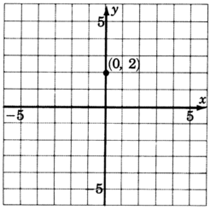2. The slope, $m$ , is $\frac{3}{4}$ . This means that if we start at any point on the line and move our pencil $3$ units up and then $4$ units to the right, we’ll be back on the line. Start at a known point, the $y\text{-intercept}\text{\hspace{0.17em}}\left(0,\text{\hspace{0.17em}}2\right)$ . Move up $3$ units, then move $4$ units to the right. Mark a point at this location. (Note also that $\frac{3}{4}=\frac{-3}{-4}$ . This means that if we start at any point on the line and move our pencil $3$ units down and $4$ units to the left , we’ll be back on the line. Note also that $\frac{3}{4}=\frac{\frac{3}{4}}{1}$ . This means that if we start at any point on the line and move to the right $1$ unit, we’ll have to move up $3/4$ unit to get back on the line.)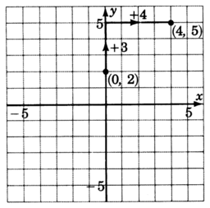3. Draw a line through both points.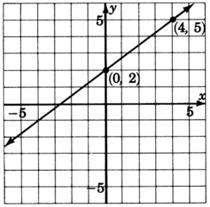$y=-\frac{1}{2}x+\frac{7}{2}$

1. The $y\text{-intercept}$ is the point $\left(0,\text{\hspace{0.17em}}\frac{7}{2}\right)$ . Thus the line crosses the $y\text{-axis}$ $\frac{7}{2}\text{\hspace{0.17em}}\text{units}$ above the origin. Mark a point at $\left(0,\text{\hspace{0.17em}}\frac{7}{2}\right)$ , or $\left(0,\text{\hspace{0.17em}}3\frac{1}{2}\right)$ .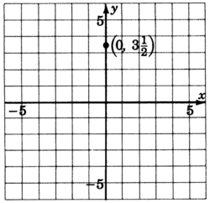2. The slope, $m$ , is $-\frac{1}{2}$ . We can write $-\frac{1}{2}$ as $\frac{-1}{2}$ . Thus, we start at a known point, the $y\text{-intercept}$ $\left(0,\text{\hspace{0.17em}}3\frac{1}{2}\right)$ , move down one unit (because of the $-1$ ), then move right $2$ units. Mark a point at this location.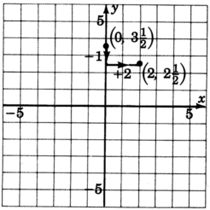3. Draw a line through both points.$y=\frac{2}{5}x$

1. We can put this equation into explicit slope-intercept by writing it as $y=\frac{2}{5}x+0$ .

The $y\text{-intercept}$ is the point $\left(0,\text{\hspace{0.17em}}0\right)$ , the origin. This line goes right through the origin.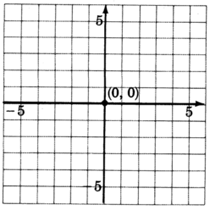2. The slope, $m$ , is $\frac{2}{5}$ . Starting at the origin, we move up $2$ units, then move to the right $5$ units. Mark a point at this location.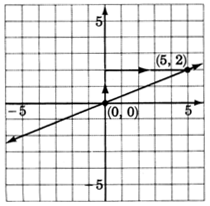3. Draw a line through the two points.

$y=2x-4$

1. The $y\text{-intercept}$ is the point $\left(0,\text{\hspace{0.17em}}-4\right)$ . Thus the line crosses the $y\text{-axis}\text{\hspace{0.17em}}4$ units below the origin. Mark a point at $\left(0,\text{\hspace{0.17em}}-4\right)$ .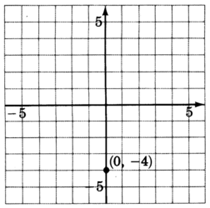2. The slope, $m$ , is 2. If we write the slope as a fraction, $2=\frac{2}{1}$ , we can read how to make the changes. Start at the known point $\left(0,\text{\hspace{0.17em}}-4\right)$ , move up $2$ units, then move right $1$ unit. Mark a point at this location.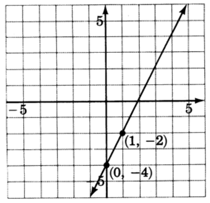3. Draw a line through the two points.

## Practice set a

Use the $y\text{-intercept}$ and the slope to graph each line.

$y=\frac{-2}{3}x+4$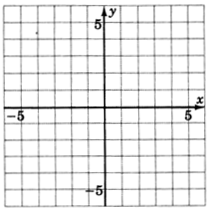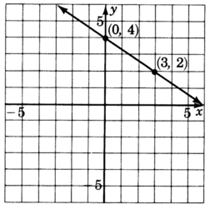$y=\frac{3}{4}x$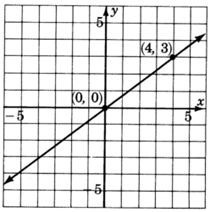## Excercises

For the following problems, graph the equations.

$y=\frac{2}{3}x+1$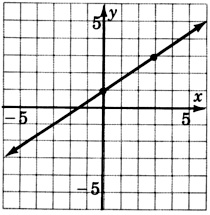$y=\frac{1}{4}x-2$$y=5x-4$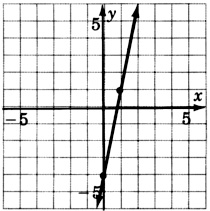$y=-\frac{6}{5}x-3$$y=\frac{3}{2}x-5$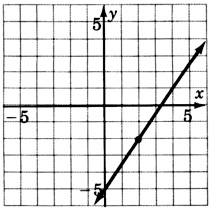$y=\frac{1}{5}x+2$$y=-\frac{8}{3}x+4$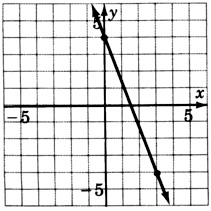$y=-\frac{10}{3}x+6$$y=1x-4$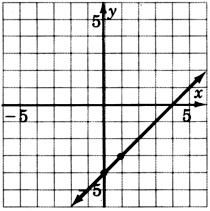$y=-2x+1$$y=x+2$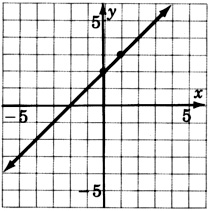$y=\frac{3}{5}x$$y=-\frac{4}{3}x$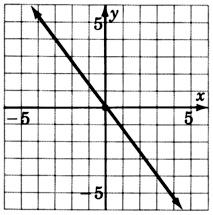$y=x$$y=-x$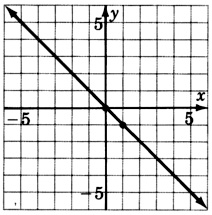$3y-2x=-3$$6x+10y=30$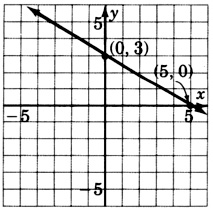$x+y=0$## Excersise for review

( [link] ) Solve the inequality $2-4x\ge x-3$ .

$x\le 1$

( [link] ) Graph the inequality $y+3>1$ .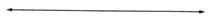( [link] ) Graph the equation $y=-2$ .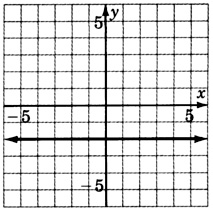( [link] ) Determine the slope and $y\text{-intercept}$ of the line $-4y-3x=16$ .

( [link] ) Find the slope of the line passing through the points $\left(-1,\text{\hspace{0.17em}}5\right)$ and $\left(2,\text{\hspace{0.17em}}3\right)$ .

$m=\frac{-2}{3}$

Preparation and Applications of Nanomaterial for Drug Delivery
Application of nanotechnology in medicine
what is variations in raman spectra for nanomaterials
I only see partial conversation and what's the question here!
what about nanotechnology for water purification
please someone correct me if I'm wrong but I think one can use nanoparticles, specially silver nanoparticles for water treatment.
Damian
yes that's correct
Professor
I think
Professor
what is the stm
is there industrial application of fullrenes. What is the method to prepare fullrene on large scale.?
Rafiq
industrial application...? mmm I think on the medical side as drug carrier, but you should go deeper on your research, I may be wrong
Damian
How we are making nano material?
what is a peer
What is meant by 'nano scale'?
What is STMs full form?
LITNING
scanning tunneling microscope
Sahil
how nano science is used for hydrophobicity
Santosh
Do u think that Graphene and Fullrene fiber can be used to make Air Plane body structure the lightest and strongest. Rafiq
Rafiq
what is differents between GO and RGO?
Mahi
what is simplest way to understand the applications of nano robots used to detect the cancer affected cell of human body.? How this robot is carried to required site of body cell.? what will be the carrier material and how can be detected that correct delivery of drug is done Rafiq
Rafiq
if virus is killing to make ARTIFICIAL DNA OF GRAPHENE FOR KILLED THE VIRUS .THIS IS OUR ASSUMPTION
Anam
analytical skills graphene is prepared to kill any type viruses .
Anam
Any one who tell me about Preparation and application of Nanomaterial for drug Delivery
Hafiz
what is Nano technology ?
write examples of Nano molecule?
Bob
The nanotechnology is as new science, to scale nanometric
brayan
nanotechnology is the study, desing, synthesis, manipulation and application of materials and functional systems through control of matter at nanoscale
Damian
Is there any normative that regulates the use of silver nanoparticles?
what king of growth are you checking .?
Renato
What fields keep nano created devices from performing or assimulating ? Magnetic fields ? Are do they assimilate ?
why we need to study biomolecules, molecular biology in nanotechnology?
?
Kyle
yes I'm doing my masters in nanotechnology, we are being studying all these domains as well..
why?
what school?
Kyle
biomolecules are e building blocks of every organics and inorganic materials.
Joe
anyone know any internet site where one can find nanotechnology papers?
research.net
kanaga
sciencedirect big data base
Ernesto
Introduction about quantum dots in nanotechnology
hi
Loga
Please keep in mind that it's not allowed to promote any social groups (whatsapp, facebook, etc...), exchange phone numbers, email addresses or ask for personal information on QuizOver's platform.ByByBy John GabrieliBy OpenStaxBy Katie MontroseBy Melinda SalzerBy Jonathan LongBy John GabrieliByBy OpenStaxBy OpenStaxBy Madison Christian